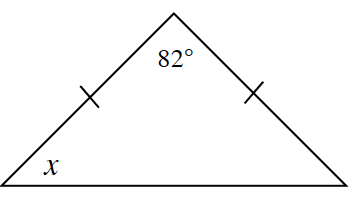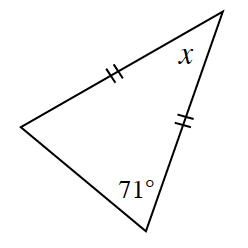### Home > GC > Chapter 7 > Lesson 7.2.1 > Problem7-52

7-52.

Use the geometric relationships in the diagrams below to solve for $x$.

1.This is an isosceles triangle. Are there any special qualities about isosceles triangles that you know?

Base angles in an isosceles triangles are congruent.

$180^\circ − 82^\circ = 98^\circ$ then take
$98^\circ ÷ 2 = 49^\circ$.
Both base angles will be $49^\circ$.

$x = 49^\circ$

1.Both base angles are $71^\circ$. See part (a) for help.